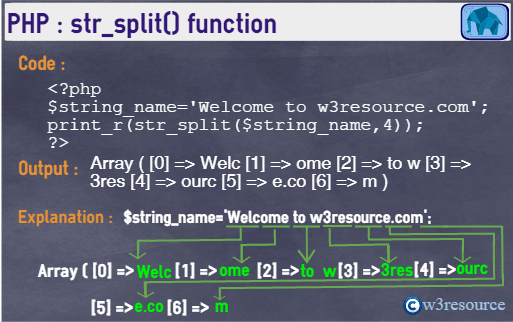# PHP: str_split() function

## Description

The str_split() function is used to convert a string to an array.

Version:

(PHP 5 )

Syntax:

`str_split(string_name, split_length)`

Parameters:

Name Description Required /
Optional
Type
string_name The string to be split. Required String
split_length The length of the chunk. Optional Integer

Return value:

If the optional split_length parameter is specified, the returned array will be broken down into chunks with each being split_length in length, otherwise, each chunk will be one character in length.
FALSE is returned if split_length is less than 1.
If the split_length length exceeds the length of the string, the entire string is returned as the first (and only) array element.

Value Type: Array.

Pictorial PresentationExample:

``````<?php
\$string_name='Welcome to w3resource.com';
print_r(str_split(\$string_name));
echo '<br>';
print_r(str_split(\$string_name,4));
echo '<br>';
?>``````

Output:

```Array  (       => W       => e       => l       => c       => o       => m       => e       =>         => t       => o       =>         => w       => 3       => r       => e       => s       => o       => u       => r       => c       => e       => .       => c       => o       => m  )
Array  (       => Welc       => ome        => to w       => 3res       => ourc       => e.co       => m  )```

View the example in the browser

PHP Function Reference

Previous: str_shuffle
Next: str_word_count

﻿

## PHP: Tips of the Day

In PHP, there are two versions of logical AND and OR operators.

Operator True if
\$a and \$b Both \$a and \$b are true
\$a && \$b Both \$a and \$b are true
\$a or \$b Either \$a or \$b is true
\$a || \$b Either \$a or \$b is true

Note that the && and || opererators have higher precedence than and and or. See table below:

Evaluation Result of \$e Evaluated as
\$e = false || true True \$e = (false || true)
\$e = false or true False (\$e = false) or true

Because of this it's safer to use && and || instead of and and or.